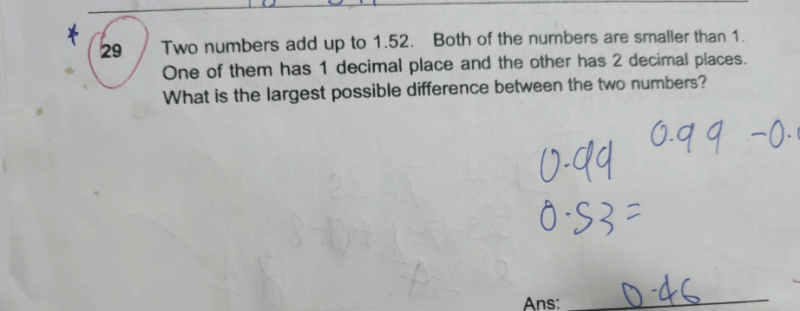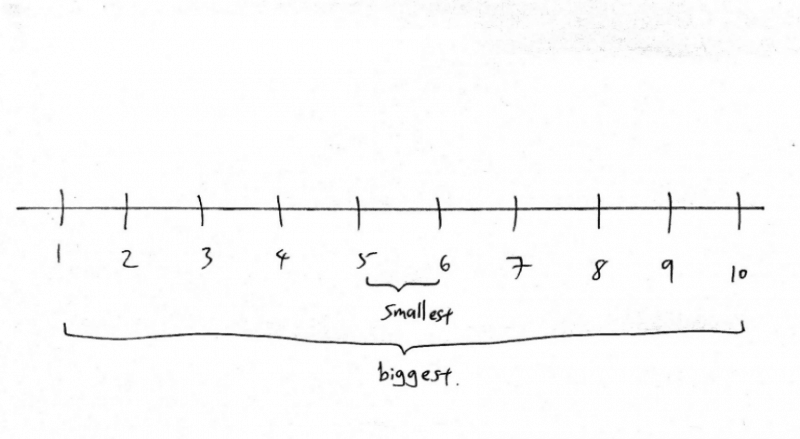# QuestionIn order to solve this question, we must understand that in order to get the largest possible difference between 2 numbers, one number must be the biggest it can possibly be, while the second number has to be the smallest it can possibly be. Take a look at the number on a number line as seen in the picture above.

If we are to pick 2 numbers from the number line (numbers 1 to 10) to give us a sum of ’11’, there will be many possible answers. We can pick 5 and 6. We can pick 4 and 7. Or perhaps 3 and 8. Or 2 and 9. And then there is 1 and 10.

We notice that the further apart these numbers are on the number line, we will be able to get the largest possible difference between them. So, if we were to pick 2 numbers from the same number line to add up to 11, and have the largest possible difference, the answer will be 1 and 10. And the difference will be 9.

So to answer the question you posted, we have to find the biggest possible number, added with the smallest possible number to get the answer of 1.52. The biggest possible number should be a 2 decimal number and not a 1 decimal number because with a 2 decimal number, i can ‘stretch out’ further, to get a bigger value. It will be 0.92. Notice that i use 0.92 because the final answer must end with x.x2 (your 1.52) in this case.

Hence the smallest number here that adds up with 0.92 to get 1.52 will be 0.6.

So my 2 numbers will be 0.92 and 0.6. And these numbers will give me the biggest possible difference.

The difference here will be 0.32.

Do PM me if you need further assistance.

www.futureachievers.com.sg

0 Replies 0 Likes